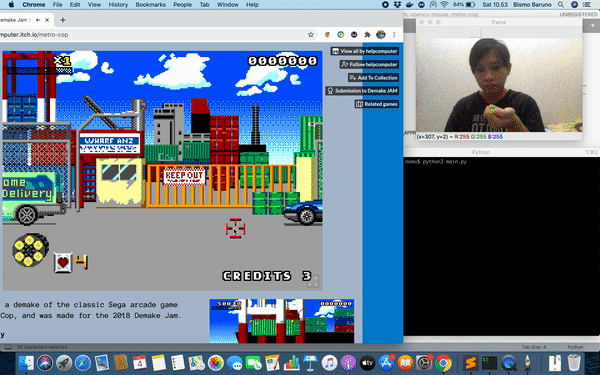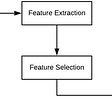# Recursion

1. If you are at home, stop moving.
2. Take one step toward home.
`Function find_max( list )              possible_max_1 = first value in list             possible_max_2 = find_max ( rest of the list );                          if ( possible_max_1 > possible_max_2 )               answer is possible_max_1             else               answer is possible_max_2             end            end`

# Parts of a Recursive Algorithm

1. Base Case (i.e., when to stop)
2. Work toward Base Case
3. Recursive Call (i.e., call ourselves)

# Matlab

`function result = add_numbers(a, b, c)            if      ( nargin() == 2 )             result = a + b;           else if ( nargin() == 3 )             result = add_numbers(a+b, c);           else             error('oops');           end                    end`

# Identify the 3 parts of the recursive algorithm:

1. Base Case: if ( nargin() == 2 ) result = a + b;
2. “Work toward base case”: a+b becomes the first parameter
3. This reduces the number of parameters (nargin) sent in to the function from 3 to 2, and 2 is the base case!

# Matlab

`% return true if we can find our way out         function success = find_way_out( maze, room )                    for every door in the room               new_room = go_through_door( maze, door )               if  ( find_way_out ( maze, new_room ) )                take that door.                 return true              end            end            % there were no ways out :(           return false          end`
`function success = find_way_out( maze, room )             if room is exit → return true             room ← mark as visited             % rest of code            ...                end`
`function success = find_way_out( maze, room )             % exit chack             if room is visited → return false             % rest of code            ...                end`

# Recursion can be equally well applied to computer algorithms:

• Summing a list of numbers:
1. Question: What is a recursive solution to summing up a list of numbers? First you should note that the sum of [1 2 3 4 5 6 7 8 9]) is equal to 1 + sum of [2 3 4 5 6 7 8 9])!
• Factorial
1. What is the definition of Factorial?
2. Answer: factorial(X) = X * (x-1) * (x-2) * … * 2
3. Hmmm, but what does “(x-1) * (x-2) * … * 2” equal?
5. What if we combine these definitions!
6. Answer 3: factorial(X) = X * factorial(X-1);
7. Lets write a recursive factorial function.
• Fibonacci
1. What is the definition of the Fibonacci number at location X in the sequence?
2. Answer: fib(x) = fib(x-1) + fib(x-2);
3. This is a recursive definition.
4. Lets write a recursive function to compute the Nth number in the Fibonacci sequence.
• Sorting
1. How can you sort a list of numbers using recursion?
2. Hint 1: Is it easier to sort a long list or a short list?
3. Hint 2: If you have two sorted lists, can you put them back together?
• Sudoku
1. How would you use a computer solve a Sudoku? How about trying every possible combination of numbers? Note: this is a brute force approach. Here is one possible “algorithm”:
2. Starting from the upper left “bucket” and moving across each row one column at a time (then down to the start of the next row, and so forth).
3. Hypothesize a valid number (what the heck, just try all 9 numbers) for the bucket. IF, you can solve the rest of the puzzle, BASED on the HYPOTHESIZED number, you have SOLVED the puzzle!
4. This is a recursive algorithm! Assume every box is assigned an index/label number (from 1 to 81):
5. Algorithm Overview Pseudocode:
• `function solve_sudoku ( current_box_id ) if all the boxes are filled return true; // solved! end // ignore already "filled" squares (assume they are correct!) if the current box is filled return the result of: solve_sudoku( next box ); end // hypothesize all 9 possible numbers for each possible number from 1 to 9 if that number is 'valid' (okay to put in box) // test row,col,square try that number in the box if you can "solve_sudoku" for the rest of the puzzle return true; // success! end end end return false; // failure end`

--

--

--

## More from Rami Louhibi

Love podcasts or audiobooks? Learn on the go with our new app.

## Day 2 Of Coding Every Day Until I Get A Job In Tech## CKAD Scenarios Playground Vim SSH## How to rename the default branch on GitHub## System Design — Different ways to implement Caching## Top 5 highest paying tech jobs in 2021 | highest paying IT jobs in 2021## Mouse Control for Shooting Game using OpenCV and Python## How Do We Help Thousands of Companies Manage Their Working Processes Efficiently?## Heading To The Future Of Contactless And Cashless Payment## Lip Reader using CNN +LSTM## Python for Machine Learning — Know the importance of Machine Learning Projects